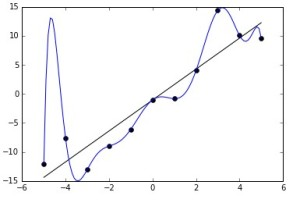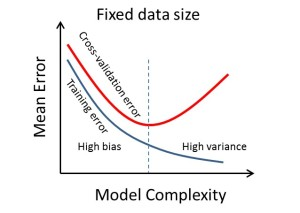# How to avoid overfitting while training?

Overfitting happens mostly because the model becomes too complex. Such a model will give poor accuracies, as it memorizes the noise in the training data. A model is usually fit by achieving the highest accuracy on the training data set. However, its efficiency is judged by its its performance on test data.

Overfitting occurs when a model begins to “memorize” training data rather than “learning” to generalize from trend.

When a model memorizes the data in its entirety, almost every point in the training data set will fit almost perfectly onto the model. But when this model is actually tested on unseen data, it will give a very poor performance.

Let us take a look at models plotted on the same set of points:The polynomial function in the blue, is heavily overfit. It passes through every point perfectly. The accuracy though will be better for the linear black line, since it closely represents the shape of the data without following the noise. Although the polynomial function is a perfect fit, the linear version can be expected to generalize better. In other words, if the two functions were used to extrapolate the data beyond the fit data, the linear function would make better predictions.

The actual data was linear, but due to noise, the points have moved a little away from the line. The complex polynomial function fails to see this as noise, but actually learns from it. Hence when we try and make a prediction, the linear curve will give a response much closer to the actual value compared to the complex polynomial function.

In any dataset, we have data which contains a certain amount of noise which occurs mainly because the data obtained is not always accurate since there maybe errors in measurement or people lying in surveys for some particular reason or because of a geographic or personal bias of the surveyor.

Let’s say we are trying to predict the marks of a student in the final exams from the mid term and continuous evaluation performances. Here we want to capture the ability of the student. But the data also contains noise such as marks obtained by cheating, or a good student not being able to perform for health reason.

The model will give good predictions if it captures the actual ability of the student while ignoring the noise. Hence in regression problems, the r squared metric is generally not preferred since it measures both the actual function and the noise in the data.

In practice it is very tough to differentiate between the two. Hence we tend to prefer models that are simpler as compared to very complex models.

How To Limit Overfitting:

We can prevent from building an overfit model using a number of techniques.

• We can use resampling to find the accuracy. The most widely used technique is k fold cross validation which divides the dataset into k folds and then tests the model k times, each time training the dataset on a different set of k-1 folds and testing it on the fold which has not been included in training. Then we calculate the average of the k accuracies obtained to give the mean cross validation accuracy.• Another method is to hold out a portion of the data, by using a random split(An 80-20 split is generally preferred with 80% of the data used for training). We keep the hold out set until the end and once the model is built we evaluate learned models using this test set. This gives us a general idea of how the model will perform on data on which we actually want to make predictions.
• We could also make the model simpler by adding a form of bias or decreasing the number of fields in the data by performing feature selection.
• Regularisation penalties like l1-regularisation or l2-regularisation can be used to make the decision surface smoother.
• Dimension reduction techniques like PCA(Principal Component Analysis) or LDA(Linear Discriminant Analysis) can be used to reduce dimensionality.
• Or, if the number of records is lesser compared to the number of features, we could always collect more data.

Take Away

We can also control the amount of overfitting of a model by using the hyperparameters. For example, in SVM, the hyper parameter C controls the amount of regularization. The C parameter trades off misclassification of training examples against simplicity of the decision surface. A low C makes the decision surface smooth, while a high C aims at classifying all training examples correctly by giving the model freedom to select more samples as support vectors. Hence a very high value of C would highly overfit the data, while a very low value of C would underfit the data. Hence we must choose an optimum value to obtain a good fit.